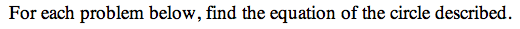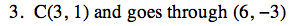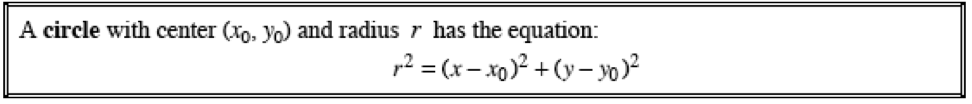13C-3.

### Missing problem for Book PCT => Chapter Ch13 => Lesson 13.1.1 => Problem 13C-3

Created from orphaned homework help problemx0 = 3y0 = 1

Use the Distance Formula to find the radius length from the center of the circle to the given point.